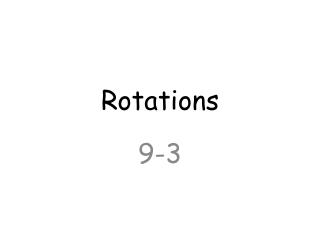DownloadDownload PresentationRotations

# Rotations

Download Presentation## Rotations

- - - - - - - - - - - - - - - - - - - - - - - - - - - E N D - - - - - - - - - - - - - - - - - - - - - - - - - - -
##### Presentation Transcript

1. Rotations 9-3

2. To describe a rotation, you need to know the center of rotation (a point), the angle of rotation (a positive number of degrees), and whether the rotation is clockwise or counterclockwise. • You can use the following two rules to rotate a figure through x° about a point R: • The image of R is itself (that is R’ = R). • For any point V, RV’ = RV and m<VRV’ = x.

3. A regular polygon has a center that is equidistant from its vertices. • Segments that connect the center to the vertices divide the polygon into congruent triangles. • A composition of rotations about the same point is itself a rotation about that point. To sketch the image, add the angles of rotation to find the total rotation.